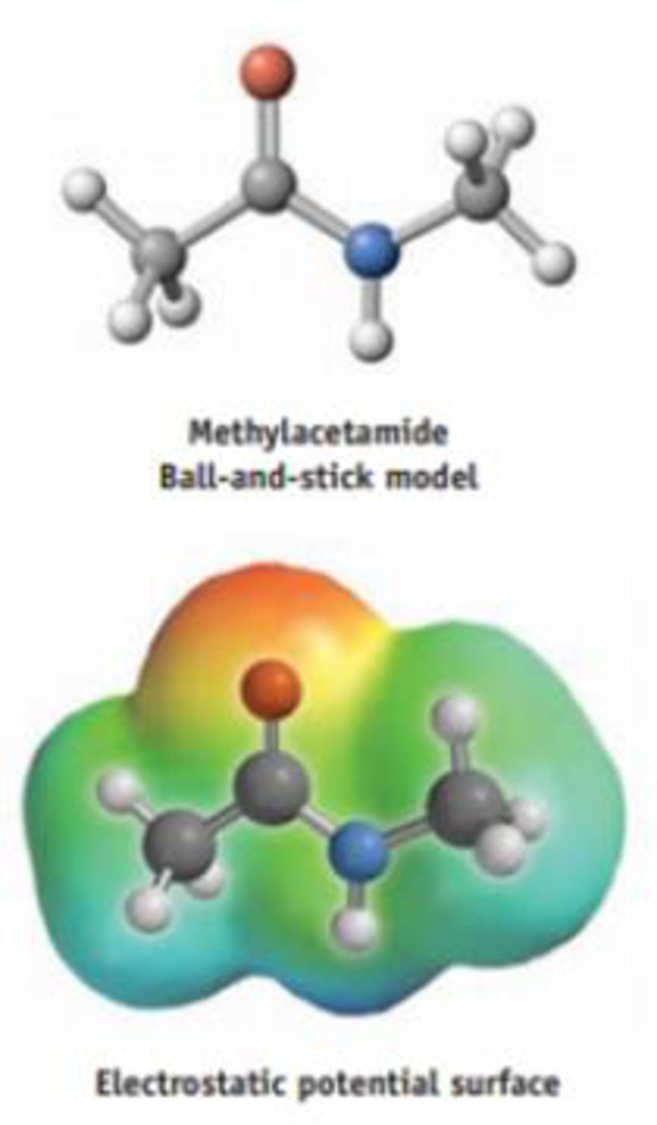Chapter 8, Problem 90IL

Chapter
Section
Textbook Problem

Methylacetamide, CH3CONHCH3, is a small molecule with an amide link (CO—NH), the group that binds one amino acid to another in proteins. (a) Is this molecule polar? (b) Where do you expect the positive and negative charges to lie in this molecule? Does the electrostatic potential surface confirm your predictions?(a)

Interpretation Introduction

Interpretation:

The molecule, methylacetamide is polar or not has to be identified.

Concept Introduction:

The unequal distribution of shared electrons caused by differences in electronegativity between bonded atoms is called bond polarity.

A molecule is non-polar, if the charge distribution is symmetric and a molecule is polar if the charge distribution is asymmetric.

I a molecule us symmetric in shape it is said to be non-polar. If a molecule is not regular, it is said to be polar.

Explanation

From the figure given below,

(b)

Interpretation Introduction

Interpretation:

The expected position of positive and negative charges in methylacetamide has to be predicted and also identify whether the electrostatic potential surface confirm the prediction.

Concept Introduction:

Electrostatic potential surface or maps are known as molecular electrical potential surfaces, that illustrates the charge distributions of molecules three dimensionally.

Still sussing out bartleby?

Check out a sample textbook solution.

See a sample solution

The Solution to Your Study Problems

Bartleby provides explanations to thousands of textbook problems written by our experts, many with advanced degrees!

Get Started

Find more solutions based on key concepts